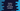# Python tutorial to calculate the sum of two string numbers## Introduction :

Finding the sum of two numbers if both numbers are integer or float, is not a problem. But what will happen if the numbers are string variable? Like ”10”, ”20” etc ? In this python tutorial, we will learn how to find the sum of two numbers if both of them are in String.

### Python program :

As mentioned above, the input numbers will be in string format. We can’t do any arithmetic operations on these string values. The program will not run. So, we will have to convert the string value to an integer for calculating the addition of any other arithmetic operation.

The conversion of string to integer is easy. Python has one inbuilt method called int() to convert a string to an integer. Just pass a string to this method and it will return you one integer value.

But it will fail if the number is not actually a number. Means, it will work for “10”, “20″, “30″ etc. which are actually numbers, but not for a string like “hello”, “hi” etc. which are not string numbers.

Let’s take a look at the following program:

``````#1
def calculateSumFor(first,second):
return int(first) + int(second)
#2
firstNumber = "100"
secondNumber = "200"
#3
print(calculateSumFor(firstNumber,secondNumber))``````

(The source code is available here):

### Explanation :

The commented numbers in the above program denote the step number below :

1. calculateSumFor() function is to calculate the sum of two numbers. These numbers should be in string format. It will return the sum of the numbers. You can see that we are converting the numbers from a string to a number using int() method.
2. Create two string variables to hold the numbers in string format: firstNumber and secondNumber.
3. Now, call the method calculateSumFor and print out the result.

If you run this code, it will look as below :

As you can see here, the sum of the two numbers is printed on the terminal. The calculation was done even though the values are strings.

If you have anything other than the number, it will throw one ValueError. Take a look at the example below :

The value of ‘firstNumber’ is “hello”, which is not a number. You should always use try-catch while using these types of conversions.

A try-catch block will add one safety check to this operation. It will first try to run the code defined inside the try block. If any exception occurred running this piece of code, it will execute all code inside the catch block.

Normally, the catch block is used to print the cause of the error. It helps us to debug easily.

``````def calculateSumFor(first,second):
try:
return int(first) + int(second)
except ValueError:
return -1
firstNumber = "hello"
secondNumber = "200"
sum = calculateSumFor(firstNumber,secondNumber)
if sum == -1:
print("Conversion failed.")
else:
print(sum)``````

Using try-catch is a good coding practice. We can avoid any runtime crash.

### Conclusion :

We have seen how to find the sum of two string numbers in python. This method is useful in many cases. e.g., if you have one server in python and you are getting numbers in string format from the client application. You can use this process to convert them. Also, always use try-catch blocks while performing any unsafe operations like this one.

Try to run the examples shown above and let me know if you have any queries.